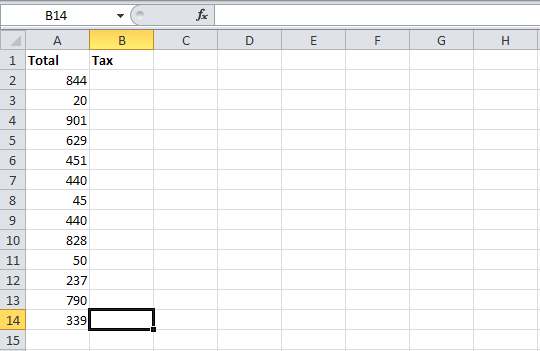# Setting Shared Formula

Contents
[ ]

## Setting Shared Formula using Aspose.Cells

Suppose you have a worksheet filled with data in the format that looks like the following sample worksheet.

Input file with one column or dataYou want to add a function in B2 that will calculate the sales tax for the first row of data. The tax is 9%. The formula that calculates the sales tax is: "=A2*0.09". This article explains how to apply this formula with Aspose.Cells.

Aspose.Cells lets you specify a formula using the Cell.Formula property. There are two options for adding formulas to the other cells (B3, B4, B5, and so on) in the column.

Either do what you did for the first cell, effectively setting the formula for each cell, updating the cell reference accordingly (A30.09, A40.09, A5*0.09 and so on). This requires the cell references for each row to be updated. It also requires Aspose.Cells to parse each formula individually, which can be time-consuming for large spreadsheets and complex formulas. It also adds extra lines of codes although loops can cut them down somewhat.

Another approach is to use a shared formula. With a shared formula, the formulas are automatically updated for the cell references in each row so that the tax would be calculated properly. The Cell.SetSharedFormula method is more efficient than the first method.

The following example demonstrates how to use it.